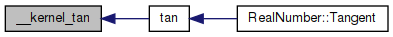amath  1.8.5 Simple command line calculator
prim.h File Reference

Primitives in math library for handling real numbers. More...

`#include "../amath.h"`
`#include "../mathr.h"`
Include dependency graph for prim.h: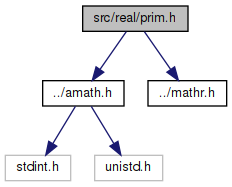This graph shows which files directly or indirectly include this file: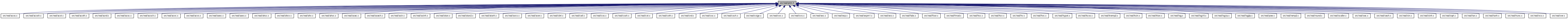Go to the source code of this file.

## Macros

#define TRIG_INEXACT(x)   ((x >= 0.0 && x < 1e-15) || (x <= 0.0 && x > -1e-15))

#define EXTRACT_WORDS(ix0, ix1, d)
Get two 32 bit ints from a double. More...

#define GET_HIGH_WORD(i, d)
Get the more significant 32 bit int from a double. More...

#define GET_LOW_WORD(i, d)
Get the less significant 32 bit int from a double. More...

#define INSERT_WORDS(d, ix0, ix1)
Set a double from two 32 bit ints. More...

#define SET_HIGH_WORD(d, v)
Set the more significant 32 bits of a double from an int. More...

#define SET_LOW_WORD(d, v)
Set the less significant 32 bits of a double from an int. More...

## Functions

double __kernel_cos (double x, double y)
Kernel cosine function. More...

double __kernel_sin (double x, double y, int iy)
Kernel sin function. More...

double __kernel_tan (double x, double y, int iy)
Kernel tan function. More...

int __kernel_rem_pio2 (double *x, double *y, int e0, int nx, int prec, const int *ipio2)
Kernel reduction function. More...

## Detailed Description

Primitives in math library for handling real numbers.

The library is based on fdlib by Sun Microsystems. The original library can be downloaded at: http://www.netlib.org/fdlibm/

or from mirror site: http://www.hensa.ac.uk/

Definition in file prim.h.

## ◆ EXTRACT_WORDS

 #define EXTRACT_WORDS ( ix0, ix1, d )
Value:
do { \
ieee_double_shape_type ew_u; \
ew_u.value = (d); \
(ix0) = ew_u.parts.msw; \
(ix1) = ew_u.parts.lsw; \
} while (0)

Get two 32 bit ints from a double.

Definition at line 156 of file prim.h.

## ◆ GET_HIGH_WORD

 #define GET_HIGH_WORD ( i, d )
Value:
do { \
ieee_double_shape_type gh_u; \
gh_u.value = (d); \
(i) = gh_u.parts.msw; \
} while (0)

Get the more significant 32 bit int from a double.

Definition at line 167 of file prim.h.

## ◆ GET_LOW_WORD

 #define GET_LOW_WORD ( i, d )
Value:
do { \
ieee_double_shape_type gl_u; \
gl_u.value = (d); \
(i) = gl_u.parts.lsw; \
} while (0)

Get the less significant 32 bit int from a double.

Definition at line 177 of file prim.h.

## ◆ INSERT_WORDS

 #define INSERT_WORDS ( d, ix0, ix1 )
Value:
do { \
ieee_double_shape_type iw_u; \
iw_u.parts.msw = (ix0); \
iw_u.parts.lsw = (ix1); \
(d) = iw_u.value; \
} while (0)

Set a double from two 32 bit ints.

Definition at line 187 of file prim.h.

## ◆ SET_HIGH_WORD

 #define SET_HIGH_WORD ( d, v )
Value:
do { \
ieee_double_shape_type sh_u; \
sh_u.value = (d); \
sh_u.parts.msw = (v); \
(d) = sh_u.value; \
} while (0)

Set the more significant 32 bits of a double from an int.

Definition at line 198 of file prim.h.

## ◆ SET_LOW_WORD

 #define SET_LOW_WORD ( d, v )
Value:
do { \
ieee_double_shape_type sl_u; \
sl_u.value = (d); \
sl_u.parts.lsw = (v); \
(d) = sl_u.value; \
} while (0)

Set the less significant 32 bits of a double from an int.

Definition at line 209 of file prim.h.

## ◆ TRIG_INEXACT

 #define TRIG_INEXACT ( x ) ((x >= 0.0 && x < 1e-15) || (x <= 0.0 && x > -1e-15))

Definition at line 48 of file prim.h.

## ◆ __kernel_cos()

 double __kernel_cos ( double x, double y )

Kernel cosine function.

```Kernel cos function on [-pi/4, pi/4], pi/4 ~ 0.785398164
Input x is assumed to be bounded by ~pi/4 in magnitude.
Input y is the tail of x.```
```Algorithm
1. Since cos(-x) = cos(x), we need only to consider positive x.
2. if x < 2^-27 (hx<0x3e400000 0), return 1 with inexact if x!=0.
3. cos(x) is approximated by a polynomial of degree 14 on
[0,pi/4]
4            14
cos(x) ~ 1 - x*x/2 + C1*x + ... + C6*x
where the Remes error is```
```    |              2     4     6     8     10    12     14 |     -58
|cos(x)-(1-.5*x +C1*x +C2*x +C3*x +C4*x +C5*x  +C6*x  )| <= 2
|                                      |
4     6     8     10    12     14

4. let r = C1*x +C2*x +C3*x +C4*x +C5*x  +C6*x  , then
cos(x) = 1 - x*x/2 + r
since cos(x+y) ~ cos(x) - sin(x)*y
~ cos(x) - x*y,
a correction term is necessary in cos(x) and hence
cos(x+y) = 1 - (x*x/2 - (r - x*y))
For better accuracy when x > 0.3, let qx = |x|/4 with
the last 32 bits mask off, and if x > 0.78125, let qx = 0.28125.
Then
cos(x+y) = (1-qx) - ((x*x/2-qx) - (r-x*y)).
Note that 1-qx and (x*x/2-qx) is EXACT here, and the
magnitude of the latter is at least a quarter of x*x/2,
thus, reducing the rounding error in the subtraction.
```

Definition at line 94 of file kcos.c.

References C1, C2, C3, C4, C5, C6, and one.

Referenced by cos(), and sin().

95 {
96  double a, hz, z, r, qx;
97  int32_t ix;
98
99  GET_HIGH_WORD(ix, x);
100  ix &= 0x7FFFFFFF;
101
102  // if x < 2**27
103  if (ix < 0x3E400000)
104  {
105  // generate inexact
106  if ((int)x == 0)
107  {
108  return one;
109  }
110  }
111
112  z = x * x;
113  r = z * (C1 + z * (C2 + z * (C3 + z * (C4 + z * (C5 + z * C6)))));
114
115  // |x| < 0.3
116  if (ix < 0x3FD33333)
117  {
118  return one - (0.5 * z - (z * r - x * y));
119  }
120
121  // x > 0.78125
122  if (ix > 0x3FE90000)
123  {
124  qx = 0.28125;
125  }
126  else
127  {
128  INSERT_WORDS(qx, ix - 0x00200000, 0);
129  }
130
131  hz = 0.5 * z - qx;
132  a = one - qx;
133  return a - (hz - (z * r - x * y));
134 }
#define INSERT_WORDS(d, ix0, ix1)
Set a double from two 32 bit ints.
Definition: prim.h:187
#define GET_HIGH_WORD(i, d)
Get the more significant 32 bit int from a double.
Definition: prim.h:167
static const double C1
Definition: kcos.c:50
static const double C5
Definition: kcos.c:54
static const double C6
Definition: kcos.c:55
static const double C3
Definition: kcos.c:52
static const double one
Definition: kcos.c:49
static const double C4
Definition: kcos.c:53
static const double C2
Definition: kcos.c:51
Here is the caller graph for this function: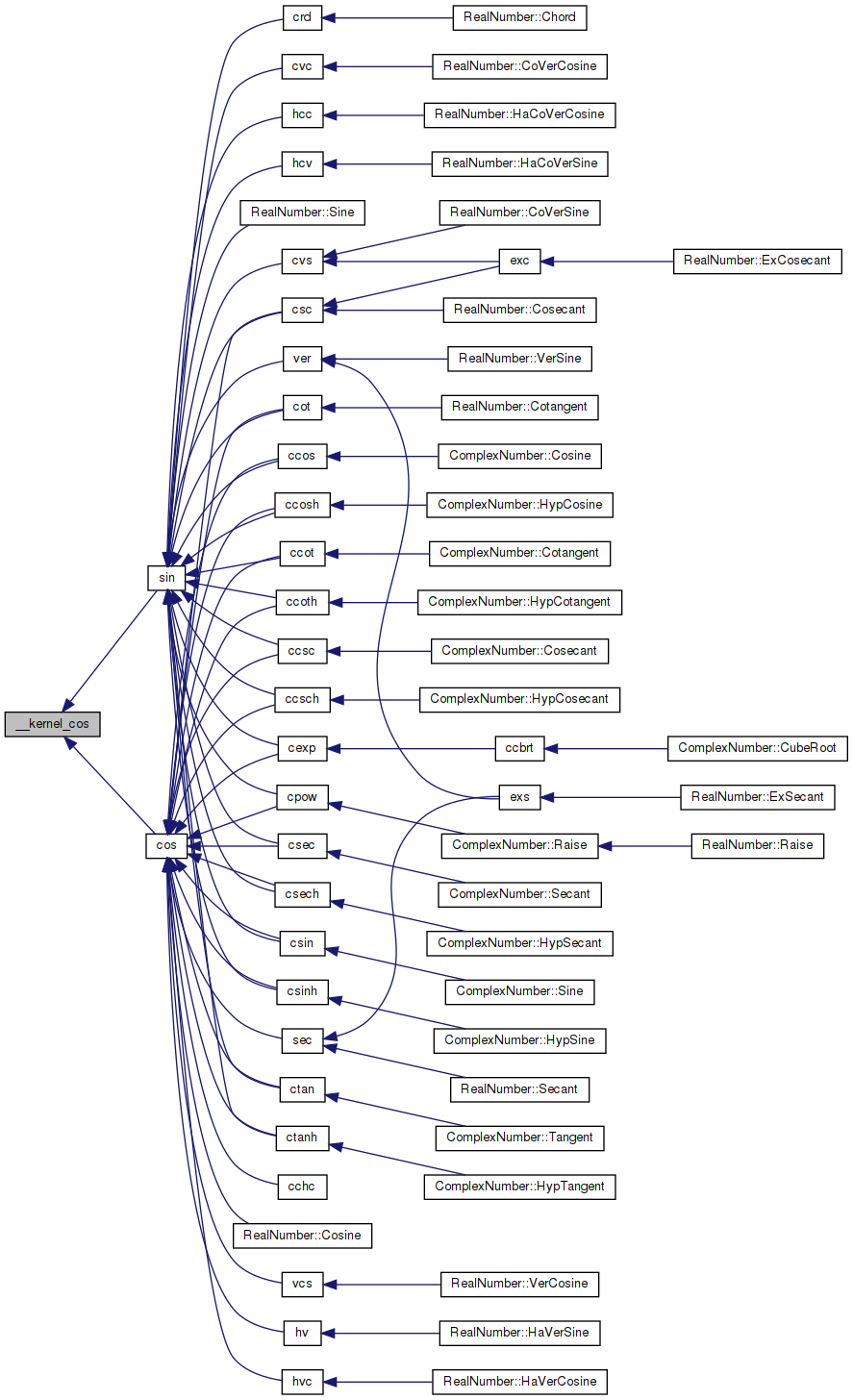## ◆ __kernel_rem_pio2()

 int __kernel_rem_pio2 ( double * x, double * y, int e0, int nx, int prec, const int * ipio2 )

Kernel reduction function.

```__kernel_rem_pio2(x,y,e0,nx,prec,ipio2)
double x[],y[]; int e0,nx,prec; int ipio2[];```
```__kernel_rem_pio2 return the last three digits of N with
y = x - N*pi/2
so that |y| < pi/2.```
```The method is to compute the integer (mod 8) and fraction parts of
(2/pi)*x without doing the full multiplication. In general we
skip the part of the product that are known to be a huge integer (
more accurately, = 0 mod 8 ). Thus the number of operations are
independent of the exponent of the input.```
`(2/pi) is represented by an array of 24-bit integers in ipio2[].`
```Input parameters:
x[] The input value (must be positive) is broken into nx
pieces of 24-bit integers in double precision format.
x[i] will be the i-th 24 bit of x. The scaled exponent
of x is given in input parameter e0 (i.e., x*2^e0
match x's up to 24 bits.```
```    Example of breaking a double positive z into x+x+x:
e0 = ilogb(z)-23
z  = scalbn(z,-e0)
for i = 0,1,2
x[i] = floor(z)
z    = (z-x[i])*2**24```
``` y[]    ouput result in an array of double precision numbers.
The dimension of y[] is:
24-bit  precision   1
53-bit  precision   2
64-bit  precision   2
113-bit precision   3
The actual value is the sum of them. Thus for 113-bit
precison, one may have to do something like:```
```    long double t,w,r_head, r_tail;
t = (long double)y + (long double)y;
w = (long double)y;
r_tail = w - (r_head - t);```
` e0 The exponent of x`
` nx dimension of x[]`
```    prec    an integer indicating the precision:
0   24  bits (single)
1   53  bits (double)
2   64  bits (extended)
``` ipio2[]
integer array, contains the (24*i)-th to (24*i+23)-th
bit of 2/pi after binary point. The corresponding
floating value is
ipio2[i] * 2^(-24(i+1)).
```
```External function:
double scalbn(), floor();```
```Here is the description of some local variables:
jk  jk+1 is the initial number of terms of ipio2[] needed
in the computation. The recommended value is 2,3,4,
6 for single, double, extended,and quad.

jz  local integer variable indicating the number of
terms of ipio2[] used.
```
` jx nx - 1`
``` jv index for pointing to the suitable ipio2[] for the
computation. In general, we want
( 2^e0*x * ipio2[jv-1]*2^(-24jv) )/8
is an integer. Thus
e0-3-24*jv >= 0 or (e0-3)/24 >= jv
Hence jv = max(0,(e0-3)/24).```
` jp jp+1 is the number of terms in PIo2[] needed, jp = jk.`
```    q[] double array with integral value, representing the
24-bits chunk of the product of x and 2/pi.```
``` q0 the corresponding exponent of q. Note that the
exponent for q[i] would be q0-24*i.```
``` PIo2[] double precision array, obtained by cutting pi/2
into 24 bits chunks.```
` f[]    ipio2[] in floating point`
` iq[]   integer array by breaking up q[] in 24-bits chunk.`
` fq[]   final product of x*(2/pi) in fq,..,fq[jk]`
``` ih integer. If >0 it indicates q[] is >= 0.5, hence
it also indicates the sign of the result.
```

Definition at line 184 of file kremp2.c.

References floor(), init_jk, one, PIo2, scalbn(), two24, twon24, and zero.

Referenced by rempio2().

185 {
186  int jz,jx,jv,jp,jk,carry,n,iq,i,j,k,m,q0,ih;
187  double z,fw,f,fq,q;
188
189  /* initialize jk*/
190  jk = init_jk[prec];
191  jp = jk;
192
193  /* determine jx,jv,q0, note that 3>q0 */
194  jx = nx-1;
195  jv = (e0-3)/24;
196  if(jv<0) jv=0;
197  q0 = e0-24*(jv+1);
198
199  /* set up f to f[jx+jk] where f[jx+jk] = ipio2[jv+jk] */
200  j = jv-jx;
201  m = jx+jk;
202  for(i=0; i<=m; i++,j++) f[i] = (j<0)? zero : (double) ipio2[j];
203
204  /* compute q,q,...q[jk] */
205  for (i=0; i<=jk; i++) {
206  for(j=0,fw=0.0; j<=jx; j++) fw += x[j]*f[jx+i-j];
207  q[i] = fw;
208  }
209
210  jz = jk;
211 recompute:
212  /* distill q[] into iq[] reversingly */
213  for(i=0,j=jz,z=q[jz]; j>0; i++,j--) {
214  fw = (double)((int)(twon24* z));
215  iq[i] = (int)(z-two24*fw);
216  z = q[j-1]+fw;
217  }
218
219  /* compute n */
220  z = scalbn(z,q0); /* actual value of z */
221  z -= 8.0*floor(z*0.125); /* trim off integer >= 8 */
222  n = (int) z;
223  z -= (double)n;
224  ih = 0;
225  if(q0>0) { /* need iq[jz-1] to determine n */
226  i = (iq[jz-1]>>(24-q0));
227  n += i;
228  iq[jz-1] -= i<<(24-q0);
229  ih = iq[jz-1]>>(23-q0);
230  }
231  else if(q0==0) ih = iq[jz-1]>>23;
232  else if(z>=0.5) ih=2;
233
234  if(ih>0) { /* q > 0.5 */
235  n += 1;
236  carry = 0;
237  for(i=0; i<jz ; i++) { /* compute 1-q */
238  j = iq[i];
239  if(carry==0) {
240  if(j!=0) {
241  carry = 1;
242  iq[i] = 0x1000000- j;
243  }
244  } else iq[i] = 0xffffff - j;
245  }
246  if(q0>0) { /* rare case: chance is 1 in 12 */
247  switch(q0) {
248  case 1:
249  iq[jz-1] &= 0x7fffff;
250  break;
251  case 2:
252  iq[jz-1] &= 0x3fffff;
253  break;
254  }
255  }
256  if(ih==2) {
257  z = one - z;
258  if(carry!=0) z -= scalbn(one,q0);
259  }
260  }
261
262  /* check if recomputation is needed */
263  if(z==zero) {
264  j = 0;
265  for (i=jz-1; i>=jk; i--) j |= iq[i];
266  if(j==0) { /* need recomputation */
267  for(k=1; iq[jk-k]==0; k++); /* k = no. of terms needed */
268
269  for(i=jz+1; i<=jz+k; i++) { /* add q[jz+1] to q[jz+k] */
270  f[jx+i] = (double) ipio2[jv+i];
271  for(j=0,fw=0.0; j<=jx; j++) fw += x[j]*f[jx+i-j];
272  q[i] = fw;
273  }
274  jz += k;
275  goto recompute;
276  }
277  }
278
279  /* chop off zero terms */
280  if(z==0.0) {
281  jz -= 1;
282  q0 -= 24;
283  while(iq[jz]==0) {
284  jz--;
285  q0-=24;
286  }
287  } else { /* break z into 24-bit if necessary */
288  z = scalbn(z,-q0);
289  if(z>=two24) {
290  fw = (double)((int)(twon24*z));
291  iq[jz] = (int)(z-two24*fw);
292  jz += 1;
293  q0 += 24;
294  iq[jz] = (int) fw;
295  } else iq[jz] = (int) z ;
296  }
297
298  /* convert integer "bit" chunk to floating-point value */
299  fw = scalbn(one,q0);
300  for(i=jz; i>=0; i--) {
301  q[i] = fw*(double)iq[i];
302  fw*=twon24;
303  }
304
305  /* compute PIo2[0,...,jp]*q[jz,...,0] */
306  for(i=jz; i>=0; i--) {
307  for(fw=0.0,k=0; k<=jp&&k<=jz-i; k++) fw += PIo2[k]*q[i+k];
308  fq[jz-i] = fw;
309  }
310
311  /* compress fq[] into y[] */
312  switch(prec) {
313  case 0:
314  fw = 0.0;
315  for (i=jz; i>=0; i--) fw += fq[i];
316  y = (ih==0)? fw: -fw;
317  break;
318  case 1:
319  case 2:
320  fw = 0.0;
321  for (i=jz; i>=0; i--) fw += fq[i];
322  y = (ih==0)? fw: -fw;
323  fw = fq-fw;
324  for (i=1; i<=jz; i++) fw += fq[i];
325  y = (ih==0)? fw: -fw;
326  break;
327  case 3: /* painful */
328  for (i=jz; i>0; i--) {
329  fw = fq[i-1]+fq[i];
330  fq[i] += fq[i-1]-fw;
331  fq[i-1] = fw;
332  }
333  for (i=jz; i>1; i--) {
334  fw = fq[i-1]+fq[i];
335  fq[i] += fq[i-1]-fw;
336  fq[i-1] = fw;
337  }
338  for (fw=0.0,i=jz; i>=2; i--) fw += fq[i];
339  if(ih==0) {
340  y = fq;
341  y = fq;
342  y = fw;
343  } else {
344  y = -fq;
345  y = -fq;
346  y = -fw;
347  }
348  }
349  return n&7;
350 }
static const double two24
Definition: kremp2.c:72
static const double twon24
Definition: kremp2.c:73
double scalbn(double x, int n)
static const int init_jk[]
Definition: kremp2.c:56
static const double PIo2[]
Definition: kremp2.c:58
static const double zero
Definition: kremp2.c:70
static const double one
Definition: kremp2.c:71
double floor(double x)
Floor function.
Definition: floor.c:62
Here is the call graph for this function: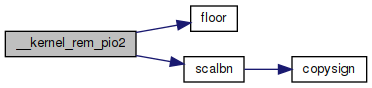Here is the caller graph for this function: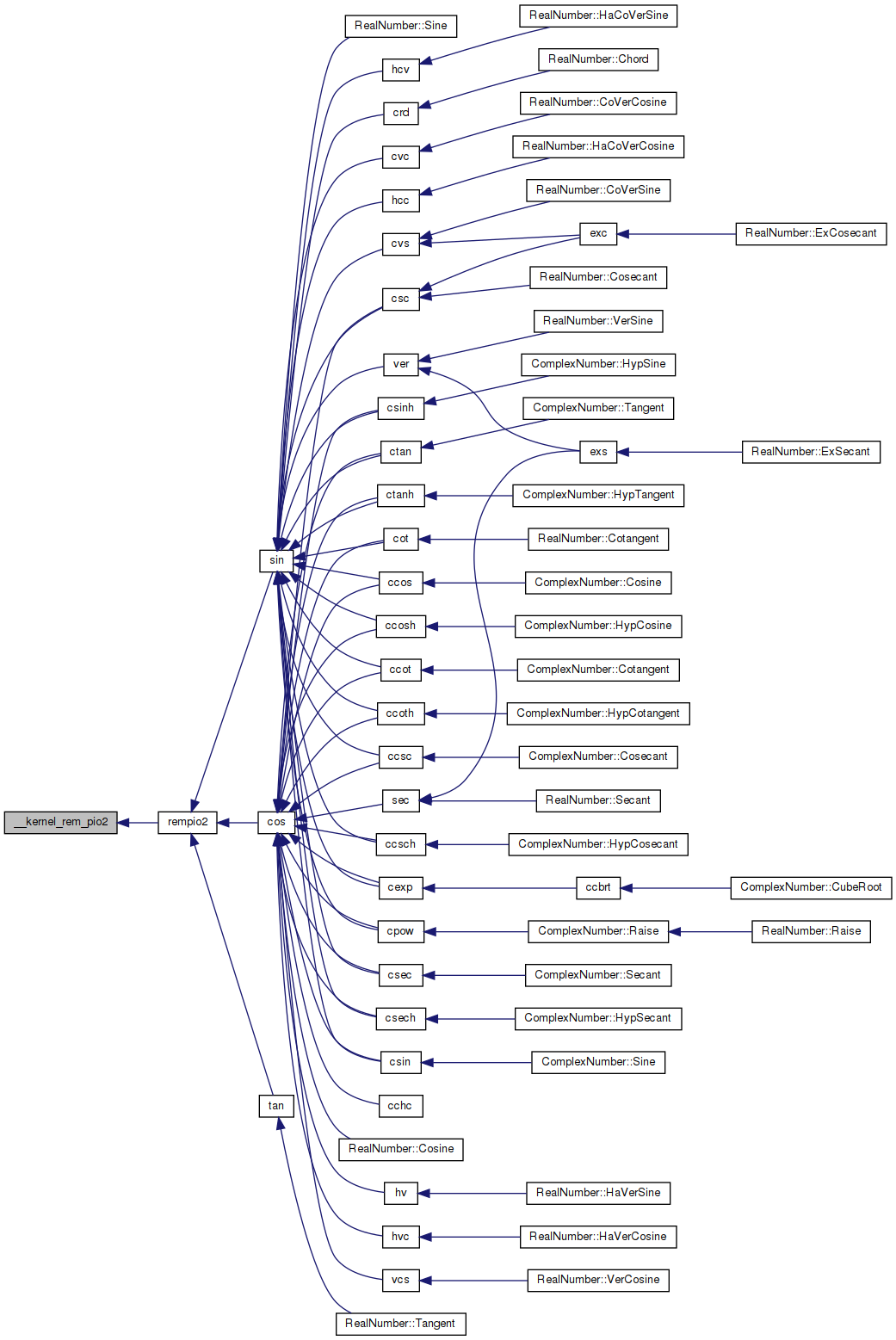## ◆ __kernel_sin()

 double __kernel_sin ( double x, double y, int iy )

Kernel sin function.

```Kernel sin function on [-pi/4, pi/4], pi/4 ~ 0.7854
Input x is assumed to be bounded by ~pi/4 in magnitude.
Input y is the tail of x.
Input iy indicates whether y is 0. (if iy=0, y assume to be 0).```
```Algorithm
1. Since sin(-x) = -sin(x), we need only to consider positive x.
2. if x < 2^-27 (hx<0X3E400000 0), return x with inexact if x!=0.
3. sin(x) is approximated by a polynomial of degree 13 on [0,pi/4]
3            13
sin(x) ~ x + S1*x + ... + S6*x```
`    where`
```    |sin(x)         2     4     6     8     10     12  |     -58
|----- - (1+S1*x +S2*x +S3*x +S4*x +S5*x  +S6*x   )| <= 2
|  x                                               |```
```  4. sin(x+y) = sin(x) + sin'(x')*y
~ sin(x) + (1-x*x/2)*y```
```       For better accuracy, let
3      2      2      2      2
r = x *(S2+x *(S3+x *(S4+x *(S5+x *S6))))
then                3    2
sin(x) = x + (S1*x + (x *(r-y/2)+y))
```

Definition at line 89 of file ksin.c.

References half, S1, S2, S3, S4, S5, and S6.

Referenced by cos(), and sin().

90 {
91  double z, r, v;
92  int32_t ix;
93
94  GET_HIGH_WORD(ix, x);
95  ix &= 0x7FFFFFFF;
96
97  // |x| < 2**-27
98  if (ix < 0x3E400000)
99  {
100  // generate inexact
101  if ((int)x == 0)
102  {
103  return x;
104  }
105  }
106
107  z = x * x;
108  v = z * x;
109  r = S2 + z * (S3 + z * (S4 + z * (S5 + z * S6)));
110
111  if (iy == 0)
112  {
113  return x + v * (S1 + z * r);
114  }
115
116  return x - ((z * (half * y - v * r) - y) - v * S1);
117 }
#define GET_HIGH_WORD(i, d)
Get the more significant 32 bit int from a double.
Definition: prim.h:167
static const double S1
Definition: ksin.c:50
static const double half
Definition: ksin.c:49
static const double S3
Definition: ksin.c:52
static const double S4
Definition: ksin.c:53
static const double S6
Definition: ksin.c:55
static const double S5
Definition: ksin.c:54
static const double S2
Definition: ksin.c:51
Here is the caller graph for this function: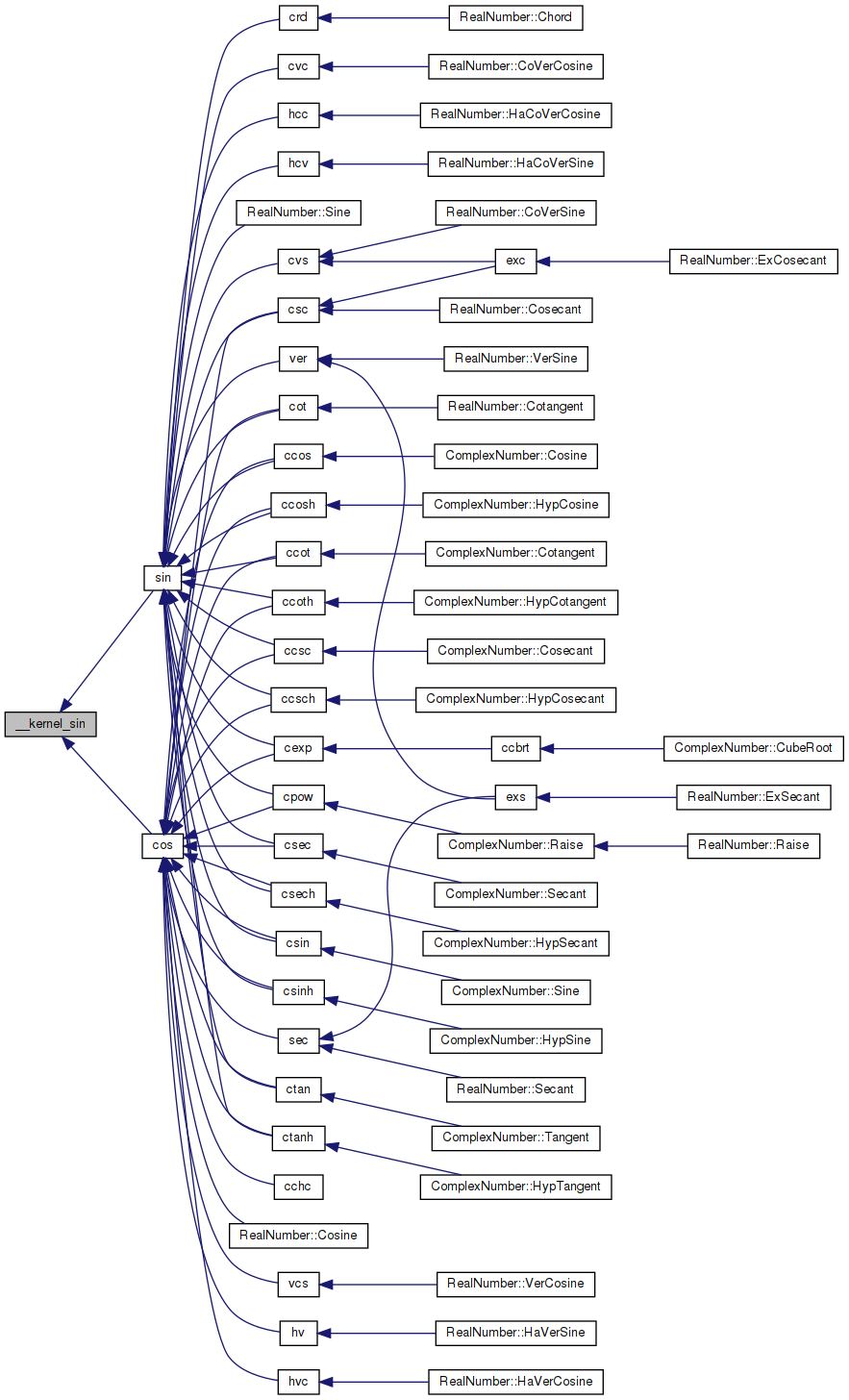## ◆ __kernel_tan()

 double __kernel_tan ( double x, double y, int iy )

Kernel tan function.

```Kernel tan function on [-pi/4, pi/4], pi/4 ~ 0.7854
Input x is assumed to be bounded by ~pi/4 in magnitude.
Input y is the tail of x.
Input k indicates whether tan (if k = 1) or -1/tan (if k = -1) is returned.```
```Algorithm
1. Since tan(-x) = -tan(x), we need only to consider positive x.
2. if x < 2^-28 (hx<0x3e300000 0), return x with inexact if x!=0.
3. tan(x) is approximated by a odd polynomial of degree 27 on
[0,0.67434]
3             27
tan(x) ~ x + T1*x + ... + T13*x
where
|tan(x)         2     4            26   |     -59.2
|----- - (1+T1*x +T2*x +.... +T13*x    )| <= 2
|  x                    |
```
```    Note: tan(x+y) = tan(x) + tan'(x)*y
~ tan(x) + (1+x*x)*y
Therefore, for better accuracy in computing tan(x+y), let
3      2      2       2       2
r = x *(T2+x *(T3+x *(...+x *(T12+x *T13))))
then
3    2
tan(x+y) = x + (T1*x + (x *(r+y)+y))```
```     4. For x in [0.67434,pi/4],  let y = pi/4 - x, then
tan(x) = tan(pi/4-y) = (1-tan(y))/(1+tan(y))
= 1 - 2*(tan(y) - (tan(y)^2)/(1+tan(y)))
```

Definition at line 108 of file ktan.c.

References fabs().

Referenced by tan().

109 {
110  double z, r, v, w, s;
111  double a, t;
112  int32_t ix, hx;
113  uint32_t low;
114
115  // high word of x
116  GET_HIGH_WORD(hx, x);
117
118  // high word of |x|
119  ix = hx & 0x7FFFFFFF;
120
121  // x < 2**-28
122  if (ix < 0x3E300000)
123  {
124  // generate inexact
125  if ((int32_t)x == 0)
126  {
127  GET_LOW_WORD(low, x);
128
129  if (((ix | low) | (iy + 1)) == 0)
130  {
131  return one / fabs(x);
132  }
133
134  if (iy == 1)
135  {
136  return x;
137  }
138
139  // compute -1 / (x+y) carefully
140  z = w = x + y;
141  SET_LOW_WORD(z, 0);
142  v = y - (z - x);
143  t = a = -one / w;
144  SET_LOW_WORD(t, 0);
145  s = one + t * z;
146  return t + a * (s + t * v);
147  }
148  }
149
150  // |x| >= 0.6744
151  if (ix >= 0x3FE59428)
152  {
153  if (hx < 0)
154  {
155  x = -x;
156  y = -y;
157  }
158  z = pio4 - x;
159  w = pio4lo - y;
160  x = z + w;
161  y = 0.0;
162  }
163
164  z = x * x;
165  w = z * z;
166
167  /*
168  * Break x^5*(T+x^2*T+...) into
169  * x^5(T+x^4*T+...+x^20*T) +
170  * x^5(x^2*(T+x^4*T+...+x^22*[T12]))
171  */
172  r = T + w * (T + w * (T + w * (T + w * (T + w * T))));
173  v = z * (T + w * (T + w * (T + w * (T + w * (T + w * T)))));
174  s = z * x;
175  r = y + z * (s * (r + v) + y);
176  r += T * s;
177  w = x + r;
178
179  if (ix >= 0x3FE59428)
180  {
181  v = (double)iy;
182  return (double)(1 - ((hx >> 30) & 2)) *
183  (v - 2.0 * (x - (w * w / (w + v) - r)));
184  }
185
186  if (iy == 1)
187  {
188  return w;
189  }
190
191  // compute -1.0 / (x+r) accurately
192  z = w;
193  SET_LOW_WORD(z, 0);
194  v = r - (z - x); // z+v = r+x
195  t = a = -1.0 / w; // a = -1.0/w
196  SET_LOW_WORD(t, 0);
197  s = 1.0 + t * z;
198  return t + a * (s + t * v);
199 }
#define pio4
Definition: ktan.c:68
#define GET_HIGH_WORD(i, d)
Get the more significant 32 bit int from a double.
Definition: prim.h:167
#define pio4lo
Definition: ktan.c:69
#define GET_LOW_WORD(i, d)
Get the less significant 32 bit int from a double.
Definition: prim.h:177
#define T
Definition: ktan.c:70
#define SET_LOW_WORD(d, v)
Set the less significant 32 bits of a double from an int.
Definition: prim.h:209
#define one
Definition: ktan.c:67
double fabs(double x)
Returns the absolute value of x.
Definition: fabs.c:51
Here is the call graph for this function: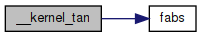Here is the caller graph for this function: